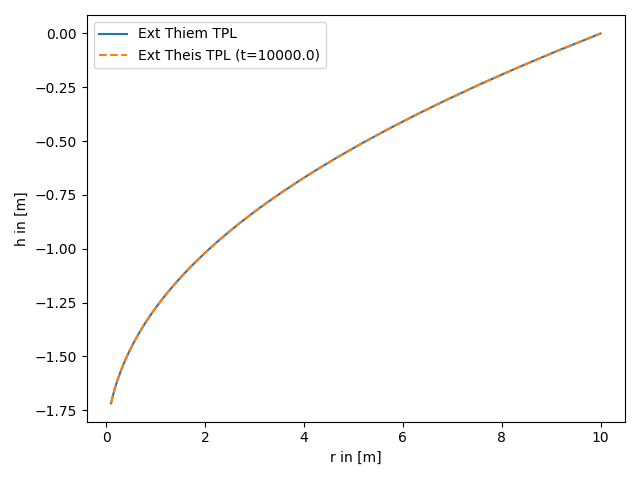# Convergence of the extended Theis solutions for truncated power laws

Here we set an outer boundary to the transient solution, so this condition coincides with the references head of the steady solution.

Reference: (not yet published)```import numpy as np
from matplotlib import pyplot as plt

from anaflow import ext_theis_tpl, ext_thiem_tpl

time = 1e4  # time point for steady state
rad = np.geomspace(0.1, 10)  # radius from the pumping well in [0, 4]
r_ref = 10.0  # reference radius

KG = 1e-4  # the geometric mean of the transmissivity
len_scale = 5.0  # correlation length of the log-transmissivity
hurst = 0.5  # hurst coefficient
var = 0.5  # variance of the log-transmissivity
dim = 1.5  # using a fractional dimension

S = 1e-4  # storativity
rate = -1e-4  # pumping rate

rad, r_ref, KG, len_scale, hurst, var, dim=dim, rate=rate
)
time, rad, S, KG, len_scale, hurst, var, dim=dim, rate=rate, r_bound=r_ref
)